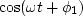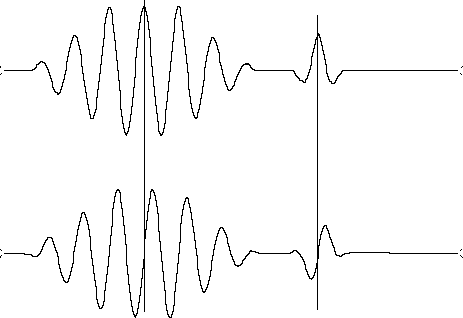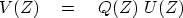Next: The analytic signal Up: HILBERT TRANSFORM Previous: A Z-transform view of

Beginning with a causal response, we switched cosines and sines in the frequency domain. Here we do so again, except that we interchange the time and frequency domains, getting a more physical interpretation.

A filter that converts sines into cosines is called aphase-shift filter" or a quadrature filter."

More specifically, if the input is,then the output should be.An example is given in Figure 2.

 hilb0 Figure 2 Input (top) filtered  with quadrature filter yields  phase-shifted signal (bottom).Let U(Z) denote the Z-transform of a real signal input and Q(Z) denote a quadrature filter. Then the output signal is(5)

Let us find the numerical values of qt. The time-derivative operation has thephase-shifting property we need. The trouble with a differentiator is that higher frequencies are amplified with respect to lower frequencies. Recall the FT and take its time derivative:(6) (7)
Thus we see that time differentiation corresponds to the weight factorin the frequency domain. The weighthas the proper phase but the wrong amplitude. The desired weight factor is(8)
where sgn is the signum'' or sign'' function. Let us transforminto the domain of sampled time t=n:(9) (10)
Examples of filtering with qn are given in Figure 2 and 3.

Since qn does not vanish for negative n, the quadrature filter is nonrealizable (that is, it requires future inputs to create its present output). If we were discussing signals in continuous time rather than sampled time, the filter would be of the form 1/t, a function that has a singularity at t=0 and whose integral over positive t is divergent. Convolution with the filter coefficients qn is therefore painful because the infinite sequence drops off slowly. Convolution with the filter qt is called Hilbert transformation."hilb
Figure 3
A Hilbert-transform pair.Next: The analytic signal Up: HILBERT TRANSFORM Previous: A Z-transform view of
Stanford Exploration Project
10/21/1998